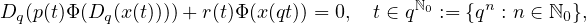Electron. J. Differential Equations, Vol. 2021 (2021), No. 50, pp. 1-23.

### Asymptotic formulas for q-regularly varying solutions of half-linear q-difference equations Katarina S. Djordjevic

Abstract:
This article studies the asymptotic behavior of positive solutions of the q-difference half-linear equationwhere q>1, Φ(x)=|x|αsgn x, α >0, p:qN0 → (0,∞), r: qN0 → R, in the framework of q-regular variation. In particular, if r is eventually of one sign, p and |r| are q-regularly varying functions such that tα+1 r(t)/p(t) → 0, as t → ∞, we obtain asymptotic formulas for the q-regularly varying solutions. Moreover, when p(t)= 1 and r is an eventually positive or eventually negative function, we obtain an asymptotic formula of a q-slowly varying solution. Using generalized regularly varying sequences, we apply these results to the half-linear difference equation case. At the end, we illustrate the obtained results with examples.

Submitted February 28, 2021. Published June 8, 2021.
Math Subject Classifications: 26A12, 39A13, 39A22.
Key Words: q-difference equation; non-oscillatory solution; asymptotic behavior; regular variation; q-regular variation; half-linear equation.

Show me the PDF file (418 KB), TEX file for this article.Katarina S. Djordjevic Department of Mathematics University of Nis, Faculty of Science and Mathematics Visegradska 33, 18000 Nis, Serbia email: katarina.kostadinov@pmf.edu.rs